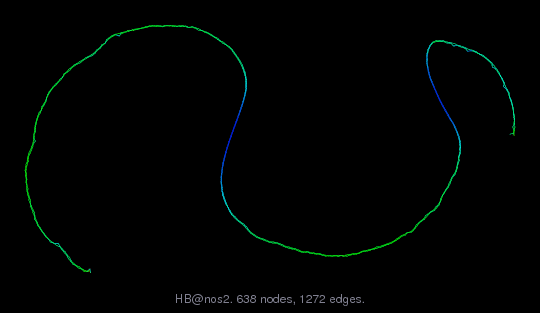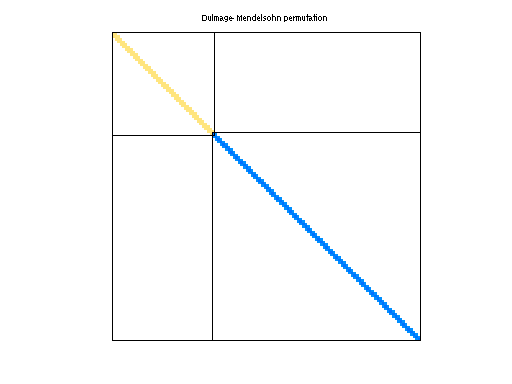Matrix: HB/nos2

Description: SYMMETRIC MATRIX, FE APPROXIMATION TO BIHARMONIC OPERATOR ON BEAM.(undirected graph drawing)• Matrix group: HB
• download as a MATLAB mat-file, file size: 6 KB. Use UFget(218) or UFget('HB/nos2') in MATLAB.

 Matrix properties number of rows 957 number of columns 957 nonzeros 4,137 structural full rank? yes structural rank 957 # of blocks from dmperm 2 # strongly connected comp. 2 explicit zero entries 0 nonzero pattern symmetry symmetric numeric value symmetry symmetric type real structure symmetric Cholesky candidate? yes positive definite? yes

 author H. Simon editor I. Duff, R. Grimes, J. Lewis date 1982 kind structural problem 2D/3D problem? yes

 Ordering statistics: result nnz(chol(P*(A+A'+s*I)*P')) with AMD 2,864 Cholesky flop count 9.2e+03 nnz(L+U), no partial pivoting, with AMD 4,771 nnz(V) for QR, upper bound nnz(L) for LU, with COLAMD 2,865 nnz(R) for QR, upper bound nnz(U) for LU, with COLAMD 4,451

 SVD-based statistics: norm(A) 1.57283e+11 min(svd(A)) 30.8423 cond(A) 5.09958e+09 rank(A) 957 sprank(A)-rank(A) 0 null space dimension 0 full numerical rank? yes

 singular values (MAT file): click here SVD method used: s = svd (full (A)) ; status: ok